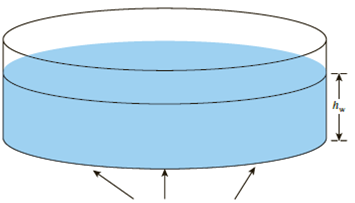Chapter 10, Problem 10.20PPrinciples of Geotechnical Enginee...

9th Edition
Braja M. Das + 1 other
ISBN: 9781305970939

Solutions

Chapter
SectionPrinciples of Geotechnical Enginee...

9th Edition
Braja M. Das + 1 other
ISBN: 9781305970939
Textbook Problem

Redo Problem 10.19 using Westergaard’s solution (Table 10.14) and compare with the solution by Boussinesq’s theory. Assume μs = 0.10.19 Figure 10.48 shows the schematic of a circular water storage facility resting on the ground surface. The radius of the storage tank, R = 2.5 m, and the maximum height of water, hw = 4 m. Determine the vertical stress increase, Δσz, at points 0, 2, 4, 8, and 10 m below the ground surface along the centerline of the tank. Use Boussinesq’s theory [Eq. (10.27)].Circular contact area of radius R on the ground surfaceFigure 10.48

To determine

Calculate the increase in vertical stress below the ground surface along the centerline of the tank using Westergaard’s solution.

Compare Westergaard’s solution by Boussinesq’s theory.

Explanation

Given information:

The radius of the storage tank (R) is 2.5m.

The maximum height of water (hw) is 4m.

The depths (z) are 0m,2m,4m,8m, and 10m.

Calculation:

Assume μs=0.

Consider the unit weight of water (γw) is 9.81kN/m3.

Calculate the intensity of pressure (q) on the circular area as shown below.

q=γwhw

Substitute 9.81kN/m3 for γw and 4m for hw.

q=9.81×4=39.24kN/m2

For the depth (z) of 2m:

Calculate the depth to radius ratio (zR) as shown below.

Substitute 2m for z and 2.5m for R.

zR=22.5=0.8

Similarly calculate the remaining values and tabulate as in Table 1.

Calculate the increase in vertical stress (Δσz) using Boussinesq’s theory as follows.

Refer Table 10.7 “Variation of Δσzq with zR” in the Text Book.

Take the value of Δσzq as 0.7562, for the value zR of 0.8.

Calculate the increase in vertical stress (Δσz) using the relation as follows.

Substitute 39.24kN/m2 for q.

Δσz39.24=0.7562Δσz=29.67kN/m2

Similarly calculate the increase in vertical stress values using Boussinesq’s theory and tabulate as in Table 1.

Show the increase in vertical stress for each depth below the center of the loaded area using Boussinesq’s theory as in Table 1.

 Radius R (m) Depth, z (m) zR Δσzq Δσz (kN/m2) 2

Still sussing out bartleby?

Check out a sample textbook solution.

See a sample solution

The Solution to Your Study Problems

Bartleby provides explanations to thousands of textbook problems written by our experts, many with advanced degrees!

Get Started

How do you display text and values in MATLAB?

Engineering Fundamentals: An Introduction to Engineering (MindTap Course List)

Would it be ethical for Amy to open such a file?

Principles of Information Security (MindTap Course List)

Describe the Big Data phenomenon.

Database Systems: Design, Implementation, & Management

Describe an ideal tack weld.

Welding: Principles and Applications (MindTap Course List)